## Quiz 26 : Ringo Rag CompanyLooking for Management Homework Help?# Quiz 26 : Ringo Rag Company

Computation of incremental or differential cost: The incremental or differential cost is the variation between the expenditure of two alternative choices, or of an increase/decrease in output levels. By extracting the information from the case of RMG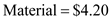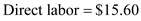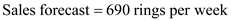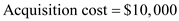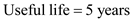Step 1 To calculate annual demand :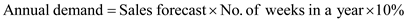Substitute: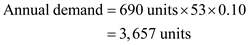Step 2 To calculate additional OH cost per 100 plastic :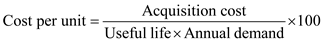Substitute: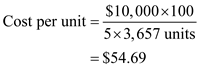Step 3 To calculate incremental cost: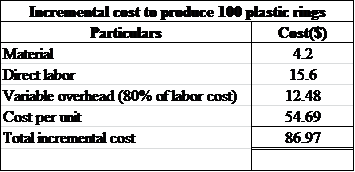Thus, the total incremental cost to produce 100 units of plastic rings is \$86.97.

Computation of incremental or differential cost of additional 34,500 units of steel rings The incremental or differential cost is the variation between the expenditure of two alternative choices, or of an increase/decrease in output levels. By extracting the information from the case of RMG Incremental cost on producing next 34,500 units of steel rings is discussed as below: With this alternative, RMG can complete the production through the summer season. Hence, the material cost would be zero because of ending inventory. The direct labor cost during this period will be 30% of the regular wages and the variable OH cost is approx 80% of direct labor costs.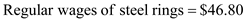To calculate the incremental cost :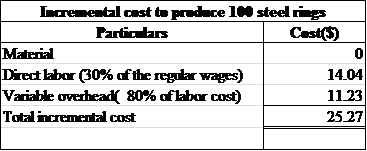Thus, the total incremental cost to produce 100 units of steel rings is \$25.27.

Computation of incremental or differential cost on ending inventory The incremental or differential cost is the variation between the expenditure of two alternative choices, or of an increase/decrease in output levels. By extracting the information from the case of RMG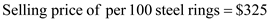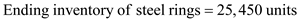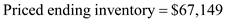Step 1 To calculate the selling price of ending inventory of steel rings: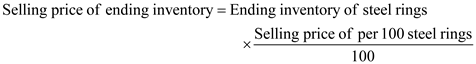Substitute: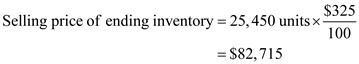Step 2 To calculate the cost differential on ending inventory of steel rings: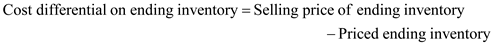Substitute: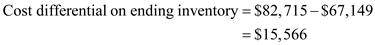Thus, the company may earned a profit of \$15,566 if it choose to sell finished steel rings that remained as ending inventory.